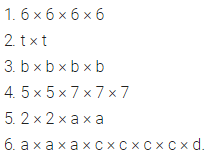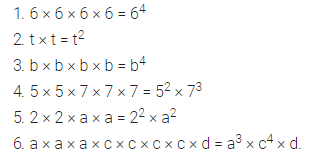# Express the following in exponential form : (1) 6 × 6 × 6 × 6 (2) t × t (3) b × b × b × b

Express the following in exponential form :